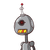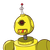# The cost of two pizzas is Rs. 198. Find the cost of 15 pizzas. ​

The cost of two pizzas is Rs. 198. Find the cost of 15 pizzas. ​

### 2 thoughts on “The cost of two pizzas is Rs. 198. Find the cost of 15 pizzas. ​”

1.15*99 = 1485

2.